# Electrochemistry Formulas for NEET

The tendency of an electrode to lose electrons is called oxidation potential, while tendency to gain electrons is called reduction potential.

EMF of a due cell is a measure of the free energy change which determines the tendency of the overall reaction to occur.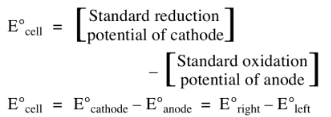If the EMF comes out to be positive, the reaction takes place.

## Nernst Equation

Dependence of electrode potential and EMF on concentration and temperature.

For electrode potential;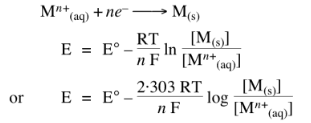For solids, liquids or gases at 1 atom.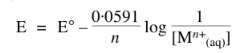## Gibbs Free Energy Change

Gibbs free energy change in an electrochemical reaction can be expressed as the equivalent of the potential difference.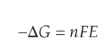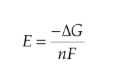## Concentration Cell

Concentration cells are electrochemical cells in which the emf arises from a transfer of material from one electrode to another due to a concentration difference between the two.

In general for concentration cells.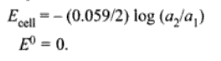## Electrolysis

The process of decomposition of an electrolyte by the passage of electricity through its aqueous solution or molten state.

Electrodes used in the electrolysis of different electrolytes are of two types, inert electrodes and active electrodes.

## Faraday First Law of Electrolysis

The mass of primary products formed at an electrode by electrolysis is directly proportional to the quantity of electricity passed.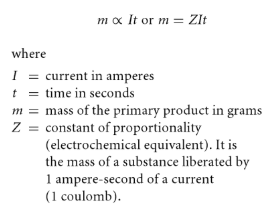## Faraday Second Law of Electrolysis

The masses of different primary products formed by equal amounts of electricity are proportional to the ratio of molar mass to the number of electrons involved with a particular reaction.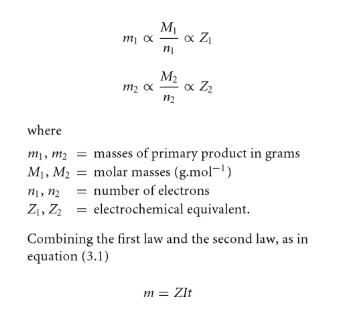## Conductance

In relation to electrolytes, the term conductance (C) is used more frequently than resistance. Conductance implies the case with which electric current can flow through a conductor. It is defined as the reciprocal of resistance.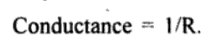### Specific Conductance

Specific conductance = Conductance x Cell constant

### Molar Conductance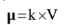### Equivalent Conductance

Equivalent conductance = (Molar conductance) / n

Where n = (Molecular mass)/(Equivalent mass)

## Kohlrausch Law

The molar conductivity of an electrolyte at infinite dilution can be expressed as the sum of the ionic conductivities of cations and anions each multiplied by the number of ions present in one formula unit of the electrolyte.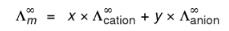## Applications of Kohlrausch Law

1. In the calculation of molar conductivity at infinite dilution for a weak electrolyte.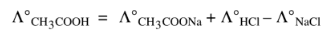2. In the calculation of the degree of dissociation.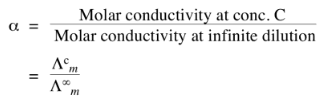3. In the calculation of solubility of a sparingly soluble salt. As the solution is saturated but infinitely dilute.

Molarity = solubility

Hence,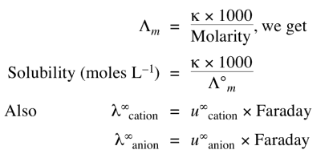Where ‘u’ are ionic mobilities at infinite dilution.

Electrochemistry is the study of chemical processes which lead to electrons moving. This movement of electrons is called electricity, which can be generated in a reaction known as an oxidation-reduction reaction by electrons from one element to another.

### Recommended Video:

Electrochemistry Class 12 – Menti Quiz | NEET Chemistry One Shot | NEET 2022 Preparation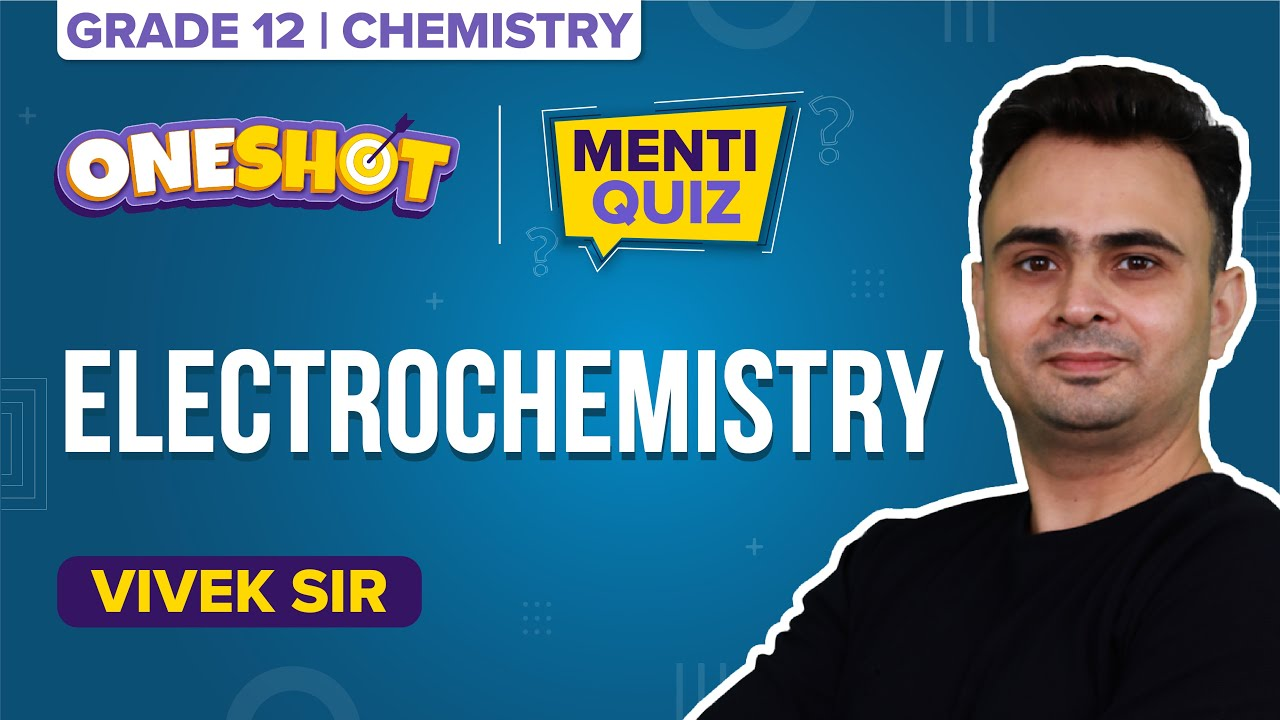Most searched posts on NEET Chemistry: